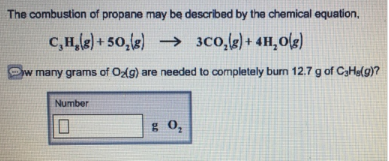# Problem: The combustion of propane may be described by the chemical equation, C3H8(g) + 5O2(g) → 3CO2(g) + 4H2O (g) w many grams of O2(g) are needed to completely burn 12.7 g of C3H8(g)?

###### FREE Expert Solution

Using the molar mass of C3H8 and O2 and their ratio from the reaction, mass of O2 needed

83% (474 ratings)###### Problem Details

The combustion of propane may be described by the chemical equation, C3H8(g) + 5O2(g) → 3CO2(g) + 4H2O (g) w many grams of O2(g) are needed to completely burn 12.7 g of C3H8(g)?